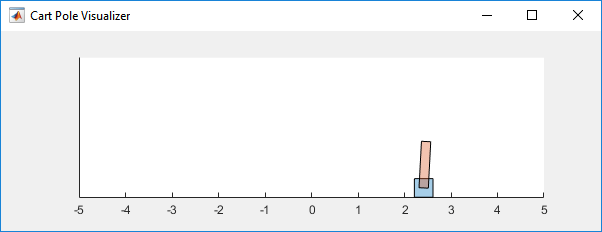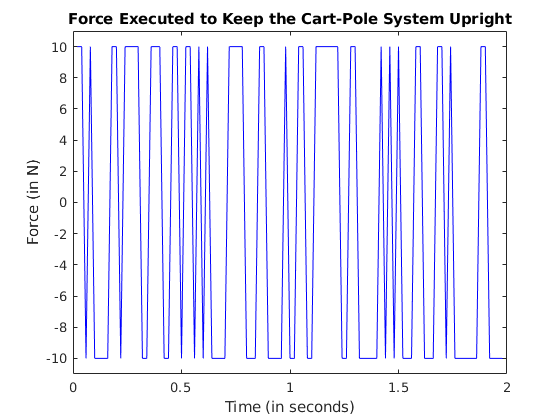# Post-Code-Generation Update of Deep Learning Network Parameters

This example shows how to incrementally update the network learnables of a deep learning network application running on edge devices such as Raspberry Pi. This example uses a cart-pole reinforcement learning application to illustrate:

1. Training a policy gradient (PG) agent to balance a cart-pole system modeled in MATLAB®. Initially, assume the agent can balance the system exerting a force in the range of -10N to 10N. For more information on PG agents, see Policy Gradient (PG) Agents (Reinforcement Learning Toolbox).

2. Generating code for the trained agent and deploying the agent on a Raspberry Pi™ target.

3. Retraining the agent in MATLAB® such that it can only exert a force of -8N to 8N.

4. Updating the learnable parameters of the deployed agent without regenerating code for the network.

### Cart-Pole MATLAB Environment

The reinforcement learning environment for this example is a pole attached to an unactuated joint on a cart, which moves along a frictionless track. The training goal is to make the pendulum stand upright without falling over.For this environment:

• The upward balanced pendulum position is `0` radians, and the downward hanging position is `pi` radians.

• The pendulum starts upright with an initial angle between –0.05 and 0.05 radians.

• The observations from the environment are the position and velocity of the cart, the pendulum angle, and the pendulum angle derivative.

• The episode terminates if the pole is more than 12 degrees from vertical or if the cart moves more than 2.4 m from the original position.

• A reward of +1 is provided for every time step that the pole remains upright. A penalty of –5 is applied when the pendulum falls.

Initialize the environment such that the force action signal from the agent to the environment is from -10 to 10 N. Later, retrain the agent so that the force action signal varies from -8N to 8N. For more information on this model, see Load Predefined Control System Environments (Reinforcement Learning Toolbox).

### Create Environment Interface

Create a predefined environment interface for the pendulum.

`env = rlPredefinedEnv("CartPole-Discrete")`
```env = CartPoleDiscreteAction with properties: Gravity: 9.8000 MassCart: 1 MassPole: 0.1000 Length: 0.5000 MaxForce: 10 Ts: 0.0200 ThetaThresholdRadians: 0.2094 XThreshold: 2.4000 RewardForNotFalling: 1 PenaltyForFalling: -5 State: [4×1 double] ```

The interface has a discrete action space where the agent can apply one of two possible force values to the cart, –10 or 10 N.

Obtain the observation and action information from the environment interface.

```obsInfo = getObservationInfo(env); numObservations = obsInfo.Dimension(1); actInfo = getActionInfo(env);```

Fix the random generator seed for reproducibility.

`rng(0)`

### Create PG Agent

The PG agent decides which action to take given observations using an actor representation. To create the actor, first create a deep neural network with one input (the observation) and one output (the action). The actor network has two outputs which correspond to the number of possible actions. For more information on creating a deep neural network policy representation, see Create Policies and Value Functions (Reinforcement Learning Toolbox).

```actorNetwork = [ featureInputLayer(numObservations,'Normalization','none','Name','state') fullyConnectedLayer(2,'Name','fc') softmaxLayer('Name','actionProb') ];```

Specify options for the actor representation using `rlRepresentationOptions` (Reinforcement Learning Toolbox).

`actorOpts = rlRepresentationOptions('LearnRate',1e-2,'GradientThreshold',1);`

Create the actor representation using the specified deep neural network and options. Specify the action and observation information for the critic, obtained from the environment interface. For more information, see `rlStochasticActorRepresentation` (Reinforcement Learning Toolbox).

`actor = rlStochasticActorRepresentation(actorNetwork,obsInfo,actInfo,'Observation',{'state'},actorOpts);`

Create the agent using the specified actor representation and the default agent options. For more information, see `rlPGAgent` (Reinforcement Learning Toolbox).

`agent = rlPGAgent(actor);`

### Train PG Agent

Train the PG agent using the following specifications:

• Run each training episode for at most 1000 episodes, with each episode lasting at most 200 time steps.

• Display the training progress in the Episode Manager dialog box (set the `Plots` option) and disable the command line display (set the `Verbose` option to `false`).

• Stop training when the agent receives an average cumulative reward greater than 195 over 100 consecutive episodes. At this point, the agent can balance the pendulum in the upright position.

For more information, see `rlTrainingOptions` (Reinforcement Learning Toolbox).

```trainOpts = rlTrainingOptions(... 'MaxEpisodes', 1000, ... 'MaxStepsPerEpisode', 200, ... 'Verbose', false, ... 'Plots','training-progress',... 'StopTrainingCriteria','AverageReward',... 'StopTrainingValue',195,... 'ScoreAveragingWindowLength',100); ```

Visualize the cart-pole system by using the `plot` function during training or simulation.

`plot(env)`The example uses a pretrained agent from the `MATLABCartpolePG.mat` MAT-file. To train the agent, set the `doTraining` flag to `true`.

```doTraining = false; if doTraining % Train the agent. trainingStats = train(agent,env,trainOpts); else % Load the pre-trained agent for the example. load('MATLABCartpolePG.mat','agent'); end```

### Generate PIL Executable for Deployment

To generate a PIL MEX function for a specified entry-point function, create a code configuration object for a static library and set the verification mode to 'PIL'. Set the target language to C++. Set the `coder.DeepLearningConfig` property of the code generation configuration object to the `coder.ARMNEONConfig` deep learning configuration object.

```cfg = coder.config('lib', 'ecoder', true); cfg.VerificationMode = 'PIL'; cfg.TargetLang = 'C++'; dlcfg = coder.DeepLearningConfig('arm-compute'); dlcfg.ArmComputeVersion = '20.02.1'; dlcfg.ArmArchitecture = 'armv7'; cfg.DeepLearningConfig = dlcfg;```

Use the MATLAB Support Package for Raspberry Pi Support Package function, `raspi` (MATLAB Support Package for Raspberry Pi Hardware), to create a connection to the Raspberry Pi. The example expects `raspi` object reuses the settings from the most recent successful connection to a Raspberry Pi board.

`r = raspi;`

Create a `coder.Hardware` object for Raspberry Pi and attach it to the code generation configuration object.

```hw = coder.hardware('Raspberry Pi'); cfg.Hardware = hw;```

### Generate PIL MEX Function for deploying PG Agent

To deploy the trained PG agent to the Raspberry Pi™ target, use the `generatePolicyFunction` (Reinforcement Learning Toolbox) command to create a policy evaluation function that selects an action based on a given observation. This command creates the `evaluatePolicy.m` file, which contains the policy function, and the `agentData.mat` file, which contains the trained deep neural network actor.

`% generatePolicyFunction(agent)`

For a given observation, the policy function evaluates a probability for each potential action using the actor network. Then, the policy function randomly selects an action based on these probabilities. In the generated `evaluatePolicy.m` file, the `actionSet` variable represents the set of possible actions that the agent can perform and is assigned the value [-10 10], based on initial conditions. However, because the example retrains the agent to exert a different force, change `actionSet` to be a runtime input to the generated `evaluatePolicy.m` file.

`type('evaluatePolicy.m')`
```function action1 = evaluatePolicy(observation1, actionSet) %#codegen % Select action from sampled probabilities probabilities = localEvaluate(observation1); % Normalize the probabilities p = probabilities(:)'/sum(probabilities); % Determine which action to take edges = min([0 cumsum(p)],1); edges(end) = 1; [~,actionIndex] = histc(rand(1,1),edges); %#ok<HISTC> action1 = actionSet(actionIndex); end %% Local Functions function probabilities = localEvaluate(observation1) persistent policy if isempty(policy) policy = coder.loadDeepLearningNetwork('agentData.mat','policy'); end observation1 = observation1(:); observation1 = dlarray(single(observation1),'CB'); probabilities = predict(policy,observation1); probabilities = extractdata(probabilities); end ```

In this example, the observation input is a four-element vector and the action input is a two-element vector.

`inputData = {ones(4,1), ones(2,1)};`

Run the codegen command to generate a PIL executable `evaluatePolicy_pil` on the host platform.

`codegen -config cfg evaluatePolicy -args inputData -report`
``` Deploying code. This may take a few minutes. ### Connectivity configuration for function 'evaluatePolicy': 'Raspberry Pi' Location of the generated elf : /home/pi/MATLAB_ws/R2022a/local-ssd/lnarasim/MATLAB/ExampleManager/lnarasim.Bdoc22a.j1840029/deeplearning_shared-ex30572827/codegen/lib/evaluatePolicy/pil Code generation successful: View report ```

#### Run Generated PIL Executable on Test Data

Load the MAT-file `experienceData.mat`. This MAT-file stores the variables `observationData` that contains sample observations for the PG agent. `observationData` contains 100 observations.

`load experienceData;`

Run the generated executable e`valuatePolicy_pil` on the observation data set.

```numActions = size(observationData, 3)-1; actions = zeros(1, numActions); actionSet = [-10; 10]; for iAction = 1:numActions actions(iAction) = evaluatePolicy_pil(observationData(:, 1, iAction), actionSet); end```
```### Starting application: 'codegen/lib/evaluatePolicy/pil/evaluatePolicy.elf' To terminate execution: clear evaluatePolicy_pil ### Launching application evaluatePolicy.elf... ```
`time = (1:numActions)*env.Ts;`

#### Plot Actions Taken by the Agent

Use a plot to visualize the output data.

```figure('Name', 'Cart-Pole System', 'NumberTitle', 'off'); plot(time, actions(:),'b-') ylim(actionSet+[-1; 1]); title("Force Executed to Keep the Cart-Pole System Upright") xlabel("Time (in seconds)") ylabel("Force (in N)")```### Retrain PG Agent

After deploying the agent, assume that the power requirement for the agent to be able to apply forces of -10N or 10N is high. One possible solution for power reduction is to retrain the agent so that it only applies force of either -8N or 8N. To retrain the agent, update the environment

`env.MaxForce = 8;`

Obtain the action information from the environment interface.

`actInfo = getActionInfo(env);`

Recreate the actor representation using the same deep neural network as before, specifying the action and observation information for the critic.

`actor = rlStochasticActorRepresentation(actorNetwork,obsInfo,actInfo,'Observation',{'state'},actorOpts);`

Create the agent using the specified actor representation and the default agent options.

`agent = rlPGAgent(actor);`

Retrain the agent in the updated environment and extract the retrained neural network from the agent.

`trainingStats = train(agent,env,trainOpts);``retrainedNet = getModel(getActor(agent));`

### Update the Deployed PG Agent on Raspberry Pi

Use the `coder.regenerateDeepLearningParameters` function to regenerate the binary files storing the network learnables based on the new values of those learnables of the network.

```codegenDirOnHost = fullfile(pwd, 'codegen/lib/evaluatePolicy'); networkFileNames = coder.regenerateDeepLearningParameters(retrainedNet, codegenDirOnHost)```
```networkFileNames = 1×2 cell {'cnn_policy0_0_fc_b.bin'} {'cnn_policy0_0_fc_w.bin'} ```

The `coder.regenerateDeepLearningParameters` function accepts the retrained deep neural network and the path to the network parameter information file emitted during code generation on the host and returns a cellarray of files containing the regenerated network learnables. Note that `coder.regenerateDeepLearningParameters `can also regenerate files containing network states, but for this example the network only has learnables. In order to update the deployed network on the Raspberry Pi device, these regenerated binary files need to be copied to the generated code folder on that board. Use the `raspi.utils.getRemoteBuildDirectory` API to find this directory. This function lists the folders of the binary files that are generated by using codegen.

```applicationDirPaths = raspi.utils.getRemoteBuildDirectory('applicationName','evaluatePolicy'); targetDirPath = applicationDirPaths{1}.directory;```

To copy the regenerated binary files, use `putFile`.

```for iFile = 1:numel(networkFileNames) putFile(r, fullfile(codegenDirOnHost, networkFileNames{iFile}), targetDirPath); end```

### Run the Executable Program on the Raspberry Pi

Re-run the generated executable `evaluatePolicy_pil` on the observation data set.

```numActions = size(observationData, 3)-1; actions = zeros(1, numActions); actionSet = [-8; 8]; for iAction = 1:numActions actions(iAction) = evaluatePolicy_pil(observationData(:, 1, iAction), actionSet); end time = (1:numActions)*env.Ts;```

#### Plot Actions Taken by the PG Agent

Use a plot to visualize the output data.

```figure('Name', 'Cart-Pole System', 'NumberTitle', 'off'); plot(time, actions(:),'b-') ylim(actionSet+[-1; 1]); title("Force Executed to Keep the Cart-Pole System Upright") xlabel("Time (in seconds)") ylabel("Force (in N)")```#### Clear PIL

`clear evaluatePolicy_pil;`
```### Host application produced the following standard output (stdout) and standard error (stderr) messages: ```